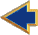### Jeremy S. DeBonet & Chris Stauffer: Using Charged Particle Systems To Simulate Fluid Flow

Resume
Research
PROJECTS

ImageMosaics
Images to ASCII
Learning PacMan
GRAPHICS

 Fluid Flow Simulation RenderMan Shaders Caustics Ray Tracing Probability Voxel Filling Multiresolution Texture Synthesis Review
Web Hacks

## PROJECT PROPOSAL

We plan to do a particle based simulation of water interaction, including water-water and water-solid interactions. By accurately modeling the attractive and repulsive forces between particles(H2O molecules and other solid particles), our simulation will exhibit such behavior as cohesion, adhesion, and wave propagation.

Most attempts at water simulation have been based on simulation of only the water surface. This however cannot fully capture the dynamic nature of water. To compensate for this, simulations have used the "hack" of adding particles when the model requires a break in the water surface. This duality is apparent in in the resulting images.

#### MODELING

Computing all the interactions between the huge numbers of molecules required to model water accurately is an intractable problem. To circumvent this problem, we will develop a number of techniques.

• By defining storing the water particles in a 3x3x3 oct-tree like data structure, we can compute only the interactions between a particle and the particles in the cells surrounding it.
This reduces the complexity from O(n2) to O(nk) where k<<n is roughly the number of particles in each of the cells in the lowest level of the 3x3x3 tree.

• By keeping a measure of the total energy of the water particles within each cell of the 3x3x3 tree and recursivly computing this total up the tree, we can determine where our computation should be spent. This will allow us to update nearly stationary particles less often, and concentrate one places where particle interactions are strong.

• We will keep some neighborhood statistics, such as flux and curl, for each cell. These will be used to alter the type of computations we perform on the particles.
For example, in neighborhoods with little or no curl it may be sufficient to simply move each particle by its velocity vector, rather than considering its interactions with other particles. This dropping the complexity down to O(n).

•For simulations of large volumes of water which in reality contain numbers of particles on the order of Avagadro's number (10.023 x 1023), we plan to instead model clusters of water molecules similar to J. Stam and E. Fiume ("Turbulent Wind Fields for Gaseous Phenomena". Proceedings of SIGGRAPH '93: 369-376.) .
This will perhaps, require new rules for particle interaction which more accurately reflect the interactions between large numbers of molecules.

#### RENDERING

Karl Sims showed an effective waterfall simulation (Particle Animation and Rendering Using Data Parallel Computation". Proceedings of GIGGRAPH '90: 405-413.) which was rendered with an accumulated raytracing algorithm. This is accomplished by passing a ray through the scene accumulating information as the ray passes through particles. The accumulated information is used to assign color and transparency. This is effective for a system where there is no global structure to the water, but it is of no use if you wish to have many of the effects associated with water(i.e. transparency, reflection, refraction, etc.).

These effects can be realized if the surface of the water is obtained. Many have accomplished this for a two-dimensional mesh(see Relevent Work Section below). A two-dimensional mesh is unrealistic, because when water is disturbed it is quite likely that it cannot be shown as a continuous surface in x and y.

We propose a method for doing this for the general case. Simply:

• Sort the particles in space(our oct-tree is already implemented)
• Move a plane through the environment. For each position of the plane:
• Create the bounding regions between different classes of particle
• Smooth the bounding regions based on particle class
• Assign relative index of refraction, color, opacity, shininess, displacement, and texture to region boundary
• Recreate a three-dimensional mesh surface using adjacent two-dimensional mesh surfaces
• Run a raytracing algorithm

This should give smooth, three-dimensional meshes around regions of particles. This makes possible the formation of artifacts like water-droplets, condensation, air bubbles in water, and turbulent surfaces. Also we can change the fluid properties to change water to blood, mercury, or coffee.

#### The Real Thing

 Figure 1.1These are two examples of the phenomena we are attempting to model.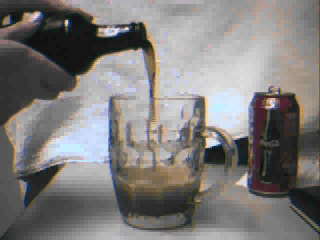1.1a The first example is an MPeg of beer being poured from a bottle into a mug.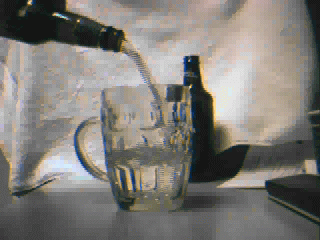1.1b The second example is water flowing from a bottle into the glass. In this example both the physical flow rate and the recording rate are faster.

#### MODELING DYNAMIC FLUID FLOW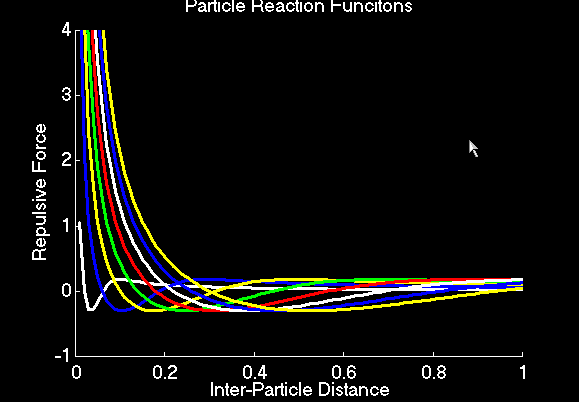Figure 1.1: Interactions between water particles are modeled with with this class of functions. The variation between curves is given by the parameter d, which is also abcissa of the zero crossing of the curve. Repulsion is given by d/x + exp ((d-x)^2/4*d^2).

#### The interactions between particles is based upon the class of curves of depicted above. A hyperbolic curve (d/x) encapsulates the repulsive van der Wells forces between groups of water molecules. A Gaussian, with mean d and standard deviation d*2 encapsulates the hydrophilic interactions which attract the molecules to one another. The parameter d is known as the principal distance, and is the distance at which two particles will be in equilibrium. The variation between curves is given by the parameter d, which is also abcissa of the zero crossing of the curve. Repulsion is given by d/x + exp ((d-x)^2/4*d^2).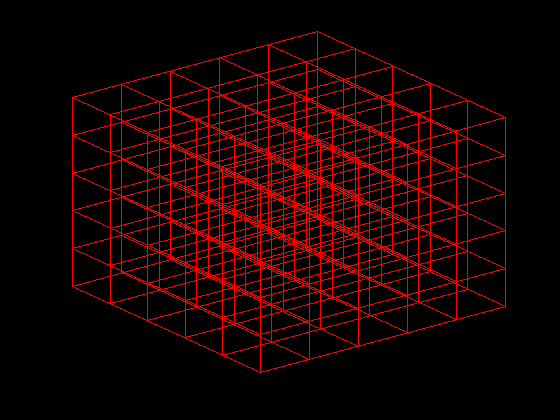Figure 1.2: To increase computational efficiency, particles were subdivided into voxel spaces. When considering inter-particle computations, only the 26 voxels which directly neighbor the voxel in which a given particle lies need be accounted for.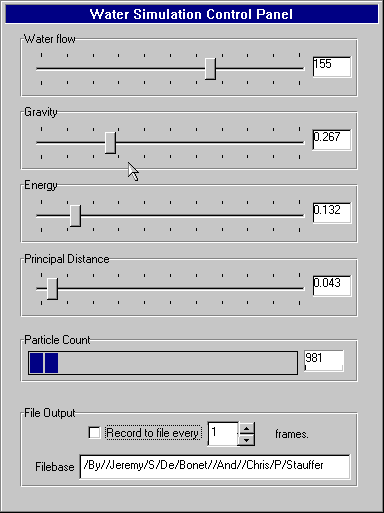Figure 1.3: The runtime IO interface to our particle simulator.

#### Interactions Between Particles and the Environment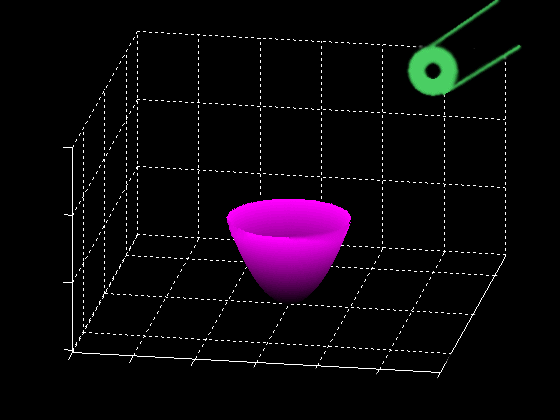Figure 1.4: An example spatial configuration, with which the liquid particles can interact. This is a model of a wineglass and variable angle winebottle source. Unlike in rendering, during particle modeling collisions with objects need to consider which side of the object they are on, and thus prevent discrete motions of the particles from allowing them to "jump" thorough non-porous surfaces.

#### Sequence 1: Wine Glass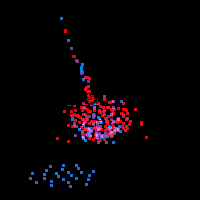Figure 1.5

A still image from our simulation of wine pouring from a bottle

#### This is a simulation of water in a kitchen sink. The stationary source will be registed to the rendering of the sink faucet. The simulation bounding box will fix the registration for the sink basin.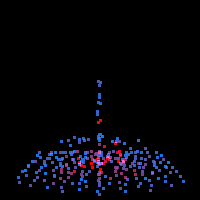Figure 1.6

A still image from our simulation of water flowing into a sink

#### Sequence 1: Wine Glass

 Figure 1.2A still image from our simulation of wine pouring from a bottle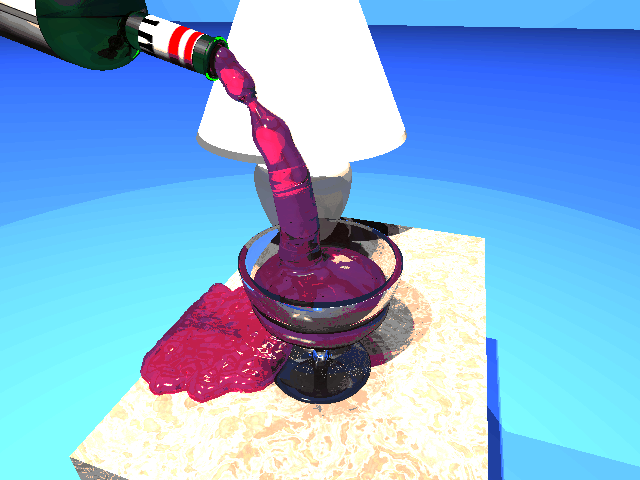#### Sequence 2: Kitchen Sink

 Figure 1.2A still image from our simulation of wine pouring from a bottle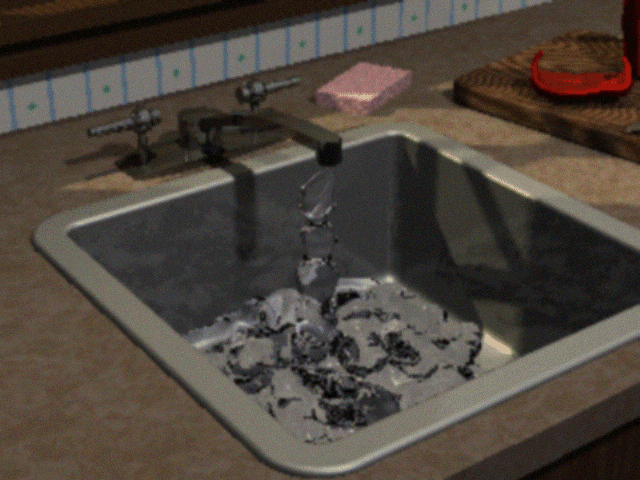#### This is a model of water in a kitchen sink. Temporal aliasing resulting from a lack of randomization of the initial particles is visible in the video. The source of the water is the faucet. The region is bounded by a box the size of the sink.

 Jeremy S. De Bonet jsd@debonet.com